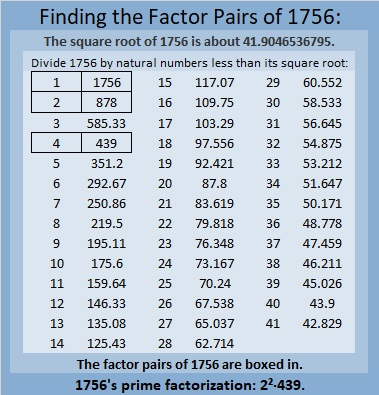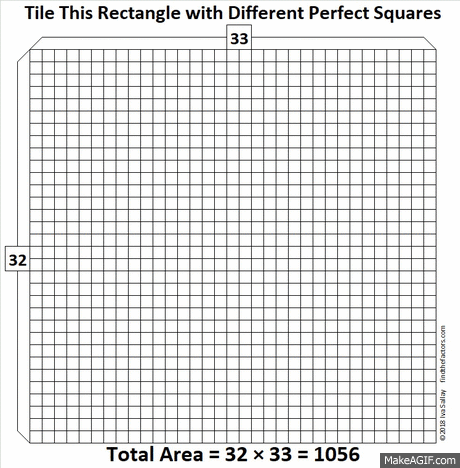# When Does 1756 Look a Little Like π?

### Today’s Puzzle:

Here are 1756 tiny squares. Solve for n and you will know which centered pentagonal number 1756 is. You could also count the pentagons from the center outward.What triangular number multiplied by 5 is one less than 1756?

How do you pronounce pentagonal? Here’s a quick video on its correct pronunciation:

Confession: I had been mispronouncing all of those terms, but not anymore!

### Factors of 1756:

• 1756 is a composite number.
• Prime factorization: 1756 = 2 × 2 × 439, which can be written 1756 = 2² × 439.
• 1756 has at least one exponent greater than 1 in its prime factorization so √1756 can be simplified. Taking the factor pair from the factor pair table below with the largest square number factor, we get √1756 = (√4)(√439) = 2√439.
• The exponents in the prime factorization are 2 and 1. Adding one to each exponent and multiplying we get (2 + 1)(1 + 1) = 3 × 2 = 6. Therefore 1756 has exactly 6 factors.
• The factors of 1756 are outlined with their factor pair partners in the graphic below.### More About the Number 1756:

The centered pentagon above doesn’t really look like π, so when does 1756 look a little bit like π? Pi day in America is next week, so this is a time-sensitive question.

Actually,1756 looks a little like 10π in base 24. You see 1756₁₀ is the same as 314₂₄.
Why? Because 3(24²) + 1(24) + 4(1) = 1756.

1756₁₀ also is the same as 1024₁₂.
Why? Because 1(12³) + 0(12²) + 2(12) + 4(1) = 1756.
I think that’s cool because 2¹º = 1024.

1756 is the difference of two squares:
440² – 438² = 1456.

# 1056 How to Tile a 32 × 33 Floor

32 × 33 = 1056, and OEIS.org informs us that those are the smallest rectangular dimensions that can be tiled with different perfect squares.

It isn’t difficult to do the tiling. All you have to remember is 32 × 33 and to put an 18 × 18 tile in a corner. The rest of the perfect square tiles seem to almost fall into place as this gif I made illustrates:make science GIFs like this at MakeaGif
Now I’ll tell you a little about the number 1056:
• 1056 is a composite number.
• Prime factorization: 1056 = 2 × 2 × 2 × 2 × 2 × 3 × 11, which can be written 1056 = 2⁵ × 3 × 11
• The exponents in the prime factorization are 5, 1, and 1. Adding one to each and multiplying we get (5 + 1)(1 + 1)(1 + 1) = 6 × 2 × 2 = 24. Therefore 1056 has exactly 24 factors.
• Factors of 1056: 1, 2, 3, 4, 6, 8, 11, 12, 16, 22, 24, 32, 33, 44, 48, 66, 88, 96, 132, 176, 264, 352, 528, 1056
• Factor pairs: 1056 = 1 × 1056, 2 × 528, 3 × 352, 4 × 264, 6 × 176, 8 × 132, 11 × 96, 12 × 88, 16 × 66, 22 × 48, 24 × 44, or 32 × 33
• Taking the factor pair with the largest square number factor, we get √1056 = (√16)(√66) = 4√66 ≈ 32.49615
Since 1056 is the product of consecutive numbers, 32 × 33, it is the sum of the first 32 even numbers:
2 + 4 + 6 + 8 + 10 + . . .  +56 + 58 + 60 + 62 + 64 = 1056